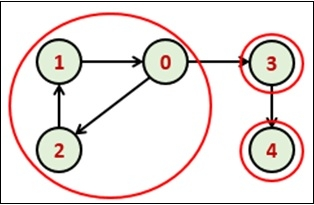# Tarjan's Algorithm for Strongly Connected Components

Tarjan’s Algorithm is used to find strongly connected components of a directed graph. It requires only one DFS traversal to implement this algorithm.Using DFS traversal we can find DFS tree of the forest. From the DFS tree, strongly connected components are found. When the root of such sub-tree is found we can display the whole subtree. That subtree is one strongly connected component.

## Input and Output

Input:
0 0 1 1 0
1 0 0 0 0
0 1 0 0 0
0 0 0 0 1
0 0 0 0 0

Output:
The strongly connected components:
4
3
1 2 0



## Algorithm

findComponent(u, disc, low, stack, stackItemFlag)

Input: The start node, discovery time, low, the disc will hold the discovery time of the vertex, and low will hold information about subtrees. The stack to hold vertices and another flag array to track which node is in the stack.

Output: Display the SCC.

Begin
time := 0      //the value of time will not be initialized for next function calls
set disc[u] := time+1 and low[u] := time + 1
time := time + 1
push u into stack
stackItemFalg[u] := true

for all vertex v which is adjacent with u, do
if v is not discovered, then
fincComponent(v, disc, low, stack, stackItemFalg)
low[u] = minimum of low[u] and low[v]
else if stackItemFalg[v] is true, then
low[u] := minimum of low[u] and disc[v]
done

poppedItem := 0
if low[u] = disc[u], then
while u is not in the stack top, do
poppedItem := top of stack
display poppedItem
stackItemFlag[poppedItem] := false
pop item from stack
done

poppedItem := top of stack
display poppedItem
stackItemFlag[poppedItem] := false
pop item from stack
End

strongConComponent(graph)

Input &,minus; The given Graph.

Output − All the strongly connected components.

Begin
initially set all items in the disc array to undiscovered
for all elements in low to φ
and mark no item is stored into the stack

for all node i in the graph, do
if disc[i] is undiscovered, then
findComponent(i, disc, low, stack, stackItemFlag)
End

## Example

#include<iostream>
#include<stack>
#define NODE 5
using namespace std;

int graph[NODE][NODE] = {
{0, 0, 1, 1, 0},
{1, 0, 0, 0, 0},
{0, 1, 0, 0, 0},
{0, 0, 0, 0, 1},
{0, 0, 0, 0, 0}
};

int min(int a, int b) {
return (a<b)?a:b;
}

void findComponent(int u, int disc[], int low[], stack<int>&stk, bool stkItem[]) {
static int time = 0;
disc[u] = low[u] = ++time;    //inilially discovery time and low value is 1
stk.push(u);
stkItem[u] = true;    //flag as u in the stack

for(int v = 0; v<NODE; v++) {
if(graph[u][v]) {
if(disc[v] == -1) {   //when v is not visited
findComponent(v, disc, low, stk, stkItem);
low[u] = min(low[u], low[v]);
} else if(stkItem[v])    //when v is in the stack, update low for u
low[u] = min(low[u], disc[v]);
}
}

int poppedItem = 0;
if(low[u] == disc[u]) {
while(stk.top() != u) {
poppedItem = stk.top();
cout << poppedItem << " ";
stkItem[poppedItem] = false;    //mark as item is popped
stk.pop();
}
poppedItem = stk.top();
cout << poppedItem <<endl;
stkItem[poppedItem] = false;
stk.pop();
}
}

void strongConComponent() {
int disc[NODE], low[NODE];
bool stkItem[NODE];
stack<int> stk;

for(int i = 0; i<NODE; i++) {    //initialize all elements
disc[i] = low[i] = -1;
stkItem[i] = false;
}

for(int i = 0; i<NODE; i++)    //initialize all elements
if(disc[i] == -1)
findComponent(i, disc, low, stk, stkItem);
}

int main() {
strongConComponent();
}

## Output

4
3
1 2 0